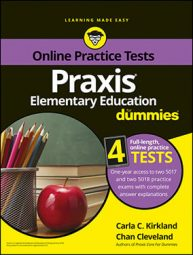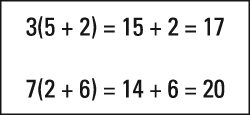##### Praxis Elementary Education For Dummies with Online Practice TestsThe Praxis Elementary Education exam contains math questions that require you to understand the four major properties of operations: commutative, associative, distributive, and substitution. The commutative and associative properties have versions for both addition and multiplication.

When three or more numbers are added, the order in which they are added does not matter. The sum will be the same no matter what the order is. That is the commutative property of addition.

8 + 2 + 7 = 2 + 7 + 8

The same principle applies to multiplication, and it's called the commutative property of multiplication.

The root of the word "commutative" is "commute," which means to move from one place to another. If a student attending classes at a college lives off campus, he commutes to class. The commutative properties are about the moving of three or more numbers when only addition or only multiplication is used.

The associative properties are like the commutative properties, but they do not pertain to changes in order. They apply to changes in the ways numbers are associated. Notice the "associate" word root in "associative." The way numbers are grouped is irrelevant when two or more numbers are added or multiplied.

The associative property of addition applies to the addition of three or more numbers, as demonstrated in this example:

(8 + 2) + 7 = 8 + (2 + 7)

The associative property of multiplication concerns the multiplication of three or more numbers:

The distributive property is the principle that multiplying a number by a sum or difference has the same result as multiplying the number by each of the numbers involved in the sum or difference and applying the other indicated operation. Portions of the value in parentheses are distributed in the multiplication process.

Think about it. If you have five sets of four marbles, you have three sets of four marbles plus another two sets of four marbles. Thus, you have twelve marbles plus another eight.

When the distributive property involves multiplying by a sum, it is distributive property of multiplication over addition. When the expression in the parentheses is a difference, the more specific name of the property is distributive property of multiplication over subtraction.

The substitution property says that if two or more number representations are equal, one can replace any of the others without changing the quantity of which the first one is part. Since 7 + 3 and 10 are equal, 5 + 10 = 5 + 7 + 3. In that example, 7 + 3 replaces 10 without causing a change in overall value because the two quantities are equal. One works as a substitute for the other.

## Practice question

1. A student wrote the following equations in a class assignment. Which of the following does the student need to be retaught?A. the distributive property B. factoring C. exponents D. unit rates